Python

# Python String Concatenation

String concatenation means creating a new string by combining two or more string values. Many built-in methods and ‘+’ operator are used to combine string values in many programming languages. ‘+’ operator is also used in python to combine string values but it works differently than other scripting languages. In JavaScript, when a string value combines with the number value then the number value will convert automatically into the string and combines with the other string value. But if you do the same task in Python then it will generate an error because Python can’t convert the number into string automatically. Many other ways exist in Python to combine string values. This article shows how you can do string concatenation in Python in different ways.  Here, spyder3 editor is used for writing and executing the scripts of this article.

### String Concatenation using ‘+’ operator

Create a python file with the following script to check how ‘+’ operator works in Python for string concatenation. Two string values are assigned to the variables named str1 and str2. These two values are combined properly and printed. Next, one string value and numeric value are assigned to the variables named text and price. If you want to combine these values then it will generate an error by mentioning that int value can be converted into str. So, the numeric value is converted into the string value by using str() method before combining the data.

#!/usr/bin/env python3
# Define to string values
str1 = "I like "
str2 = "Programming"

# Combining a string value with another string value
combineText1 = str1+str2

# Print the combined output
print("Combining string with string:\n",combineText1)

# Define a string value
text = "The price of the book is "

# Define a number value
price = 50

# Combining a string value with a number value
combineText2 = text + "\$" + str(price)

# Print the combined output
print("\nCombining string with number:\n",combineText2)

Output:

The output is shown on the right side of the image.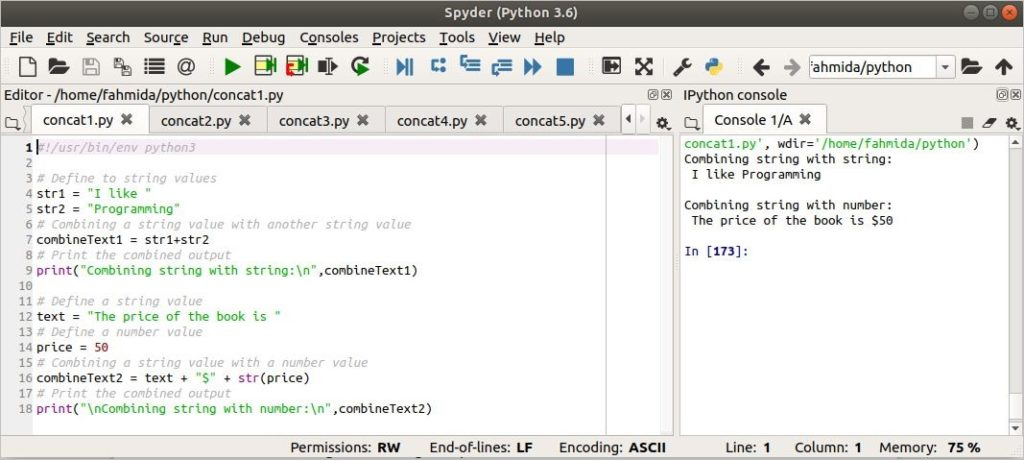### String Concatenation using ‘%’ operator

Create a python file with the following script to check how the ‘%’ symbol works for string concatenation in Python. It works like the string formatting of C language. Here, two string values are assigned in the variables, str1 and str2. You can combine two or more string values by creating a comma-separated group and using the ‘%’ symbol at the front of the group. Here, first brackets, () are used for grouping string values and ‘%s’ is used in print() method to define that, the printing values are string.

#!/usr/bin/env python3
# Define two string values
str1 = "Python"
str2 = "is a popular scripting language"

# Combine the string values using '%' operator
print("The output after combining strings:\n\n%s %s" % (str1, str2))

Output:

The output is shown on the right side of the image.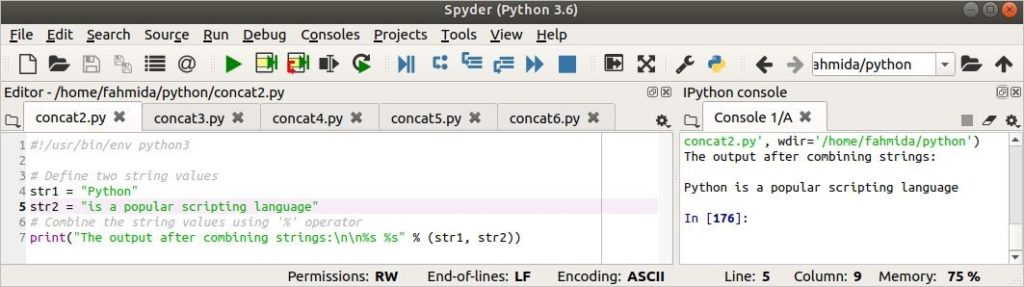### String Concatenation using format() method

If you want to combine string values more specifically then you have to use format() method of Python. Using this method, you can combine the string values based on their positions. The string positions are counted as 0,1,2 and so on. Two string values are taken from the user and assigned to the variables named str1 and str2. Next, these variables are used in the format() method as arguments. The variable positions are not mentioned in the script. So, the default variable positions are 0 and 1.

#!/usr/bin/env python3
# Define two string values
str1 = input("Enter the first string value\n")
str2 = input("Enter the second string value\n")

# Combine the string values using format() operator
combineText = "{} {}".format(str1, str2)

# Print the combined text
print("The output after combining strings:\n\n",combineText)

Output:

The output is shown on the right side of the image. Here, two input values are ‘Linux’ and ‘Hint’ and the output is ‘LinuxHint’ after the concatenation.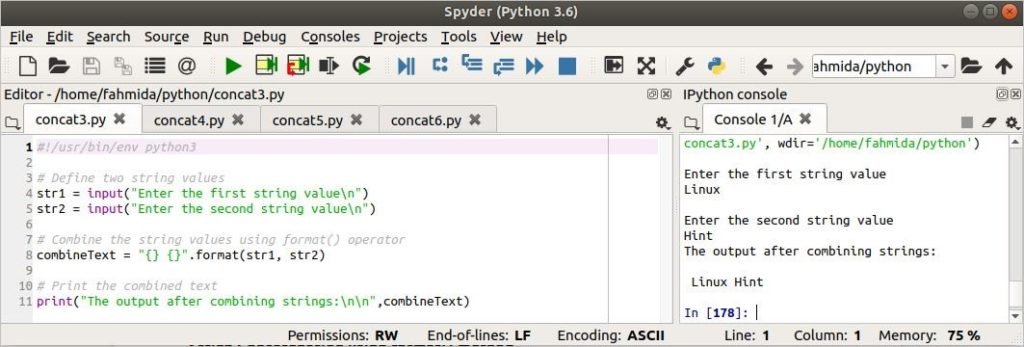### String Concatenation using join() method

join() is another useful method of Python for combining strings. If you want to add any particular string value at the time of concatenating the strings then you will require to use join() method for concatenation. Create a python file with the following script to check the use of the join() method. Three string values are assigned in the variables named str1, str2, and str3. The first join() is used to combine the strings without any specific string. The second join() is used to combine the string values with a comma(,). The third join() is used to combine the string values with a newline(\n).

#!/usr/bin/env python3
# Define two string values

str1 = "Python Programmming"
str2 = "Bash Programming"
str3 = "Java Programming"

# Using join() method to combine the strings
combineText = "".join([str1, str2, str3])

# Print the output
print("\nOutput:\n%s" % combineText)

# Using join() method with comma to combine the strings
combineText = ",".join([str1, str2, str3])

# Print the output
print("\nOutput:\n%s" % combineText)

# Using join() method with newline to combine the strings
combineText = "\n".join([str1, str2, str3])

# Print the output
print("\nOutput:\n%s" % combineText)

Output:

The output is shown on the right side of the image.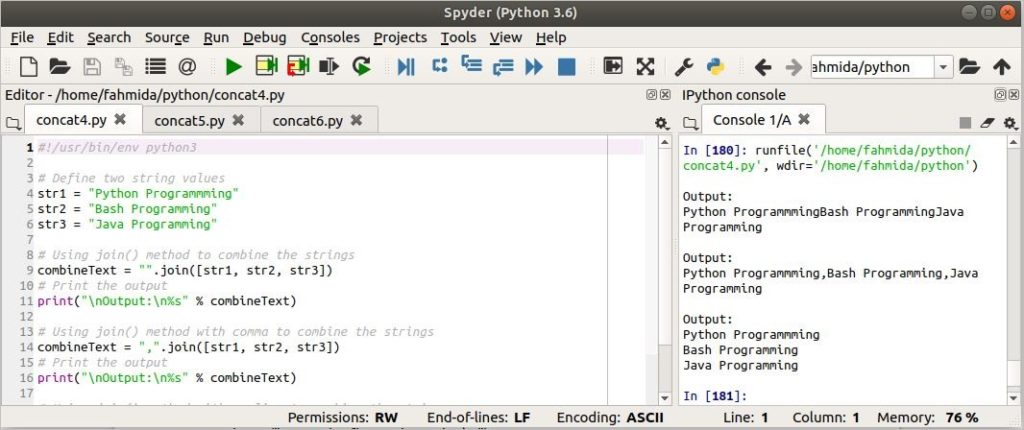### Combining the string of tuple using join() method

Create a python file with the following script. Here, join() method will combine the string values of the tuple with a newline(\n).

#!/usr/bin/env python3
# Define a tuple of string values
tupleString = ("Ubuntu","Windows", "MacOS", "Fedora","Android","RedHat")

# Combine the string values of the tuple using join() method
combineText = "\n".join(tupleString)

# Print the output
print("\nThe list of operating systems are:\n\n%s" % combineText)

Output:

The output is shown on the right side of the image.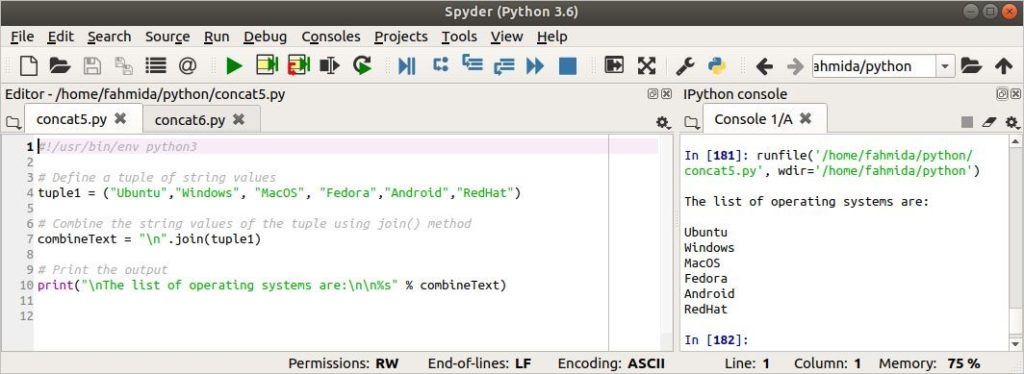### Generate a sequence of strings by combining two strings

The sequence of string values can be generated easily by using the join() method. Create a python file with the following script to create a sequence of the alphabet with a number.

#!/usr/bin/env python3
#Define two string values
str1= "ABCD "
str2 = "1 "

# Generate sequence of string using join() method
combineText = str2.join(str1)

# Print the output
print("\n%s" % combineText)

Output:

The output is shown on the right side of the image.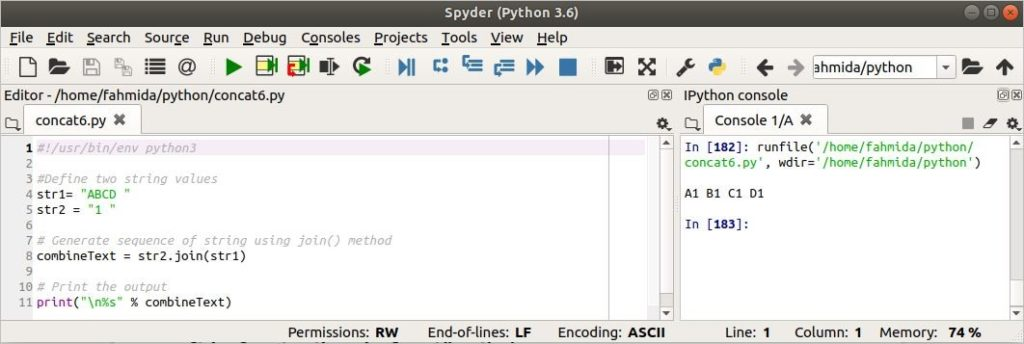### Conclusion:

Different ways of string concatenation are shown in this article by using easy examples. Hopefully, the reader will be able to combine multiple strings properly after practicing these examples.